# Make Forms and Calculator using HTML,CSS & JavaScript

hello everyone!

I like to speak about how to make different forms in using basic HTML and CSS and then how to make calculator using basic JavaScript. I have some tricky points. lets go!

In this article I like to explain makes forms using HTML, CSS and then make a calculator using JavaScript.

# First we look how to make form in HTML.

The basic HTML file structure is given below, i think you all know about basic Html.

`<DOCTYPE!> <html>   <head>   </head>   <body>        </body> </html>.`

now let’s look, how to add form in HTML. we usually use to form for getting inputs from users and get information about user inputs. so in the HTML, HTML have form element which called <form> element. so html<form> is used to create an html form for user inputs.

below the basic structure for Html form type.

`<form> elements</form>`

The form element is a container for different types of elements , attributes to create different forms. now let’s look what are the these elements, attributes and how can we get different form types from them.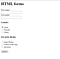Image 1-Basic form format using HTML

According to this basic form format I use several form input methods by using html. you can see text types, checkboxes, radio, submit buttons, etc. we all know every form has this kind of input methods. so now let’s look how these kind of element add in to Basic HTML form format.

In the Html forms, we use element called <input> to get inputs in different ways. input element can be displaying in many ways by using attribute now let’s look at some example.

`<input type=”text”> — — — — — use display single line text inputs.<input type=”radio”> — — — —Displays a radio button<input type=”checkbox”> — — — — — Displays a checkbox <input type=”submit”> — — — — — — Displays a submit button <input type=”button”> — — — — — — Displays a clickable button`

by using above input types we can get inputs by different ways.

now I show you the Html code of IMAGE 1 that i write.

`<doctype!><html><head><h1>HTML forms</h1></head><body><form> <label for=”fname”>First name:</label><br> <input type=”text” id=”fname” name=”fname”><br> <label for=”lname”>Last name:</label><br> <input type=”text” id=”lname” name=”lname”><h3>Gender</h3> <input type=”radio” id=”male” name=”gender” value=”male”> <label for=”male”>Male</label><br> <input type=”radio” id=”female” name=”gender” value=”female”> <label for=”female”>Female</label><br> <input type=”radio” id=”other” name=”gender” value=”other”> <label for=”other”>Other</label><h3>Favorite Books</h3> <input type=”checkbox” id=”book1" name=”book1" value=”Harry Potter”> <label for=”book11"> harry Potter</label><br> <input type=”checkbox” id=”book2" name=”book2" value=”loard of the ring”> <label for=”book2"> Loard of the ring</label><br> <input type=”checkbox” id=”book3" name=”book3" value=”the musuem”> <label for=”book3"> museum</label><br><br> <input type=”submit” value=”Submit”></form></body></html>`

According to this I had written several input types. and also in the input element you can see Id and name Attribute. Id attribute is we also know it is basic Html, and the name attribute of input element is use to submit. because when we submit the form, the value of that we enter in to form was sent by using name attribute.

and in this code you can see another Html element call <label>,

the <label> tag defines a label for many form elements. and there is attribute of label element call for. this for attribute should be equal to id attribute of the input element.

not only that we can see there are lots of Html form elements.

`<select> element — — — — — defines a drop down list<option> element — — — — — defines the option that can be selected<Textarea>element — — — — — defines multi lines input fields.<fieldset> element — — — — — — use for group related elements in a form<output> element — — — — — — define a result of a calculation<click>element — — — — define a clickable button`

# Add CSS in to HTML

we all know , we use to CSS for decorating or add some design to html files. So in here we add CSS to our html form file to improve , decorate our form format. Let’s see what the differences are when add CSS in to Html.

This is the basic html form format.

`<doctype!><html><head><h1>HTML forms</h1></head><body><form> <label for=”fname”>User name:</label><br> <input type=”text” id=”fname” name=”fname”><br> <label for=”ADD”>Address:</label><br> <input type=”text” id=”ADD” name=”ADD”><br> <label for=”DOB”>Date of Birth:</label><br> <input type=”text” id=”DOB” name=”DOB”><br> <label for=”ID”>NIC:</label><br> <input type=”text” id=”ID” name=”ID”><br> <br><label for=”country”>Country</label> <select id=”country” name=”country”> <option value=”Srilanka”>SriLanka</option> <option value=”canada”>Canada</option> <option value=”usa”>USA</option> </select><h3>marriage/unmarriage</h3> <input type=”radio” id=”marriage” name=”gender” value=”marriage”> <label for=”marriage”>Marriage</label> <input type=”radio” id=”unmarriage” name=”gender” value=”unmarriage”> <label for=”unmarriage”>unmarriage</label><h3>Gender</h3> <input type=”radio” id=”male” name=”gender” value=”male”> <label for=”male”>Male</label> <input type=”radio” id=”female” name=”gender” value=”female”> <label for=”female”>Female</label> <input type=”radio” id=”other” name=”gender” value=”other”> <label for=”other”>Other</label><br><h3><label for=”AL”>A/L Stream</label></h3> <select id=”AL” name=”AL”> <option value=”Bio Science”>Bio Science</option> <option value=”Maths”>Maths</option> <option value=”Commerce”>Commerce</option> <option value=”ART”>ART</option> </select><h3>Favorite sports</h3> <input type=”checkbox” id=”sport1" name=”sport1" value=”Cricket”><label for=”sport1"> cricket</label><br> <input type=”checkbox” id=”sport2" name=”sport2" value=”Football”> <label for=”sport2"> Football</label><br> <input type=”checkbox” id=”sport3" name=”sport3" value=”Basketball”> <label for=”sport3"> basketball</label><br><br> <input type=”submit” value=”Submit”> <input type=”button” value=”Button”><input type=”reset” value=”Reset”></form></body></html>`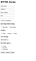this is the Html page

then we add CSS code in to html file,

`input[type=text],select { width: 60%; border: 2px solid black; padding: 12px 20px; margin: 8px 0; box-sizing: border-box; color: black;}input[type=button], input[type=submit], input[type=reset] { background-color: #4CAF50; border: none; color: white; padding: 16px 32px; text-decoration: none; margin: 4px 2px; cursor: pointer;}div { border-radius: 5px; background-color: #f2f2f2; padding: 20px;}`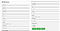this is the html file with CSS

I think you can see different.

# Make a calculator by using HTML,CSS And JavaScript

in here we can create calculator by a using this basic html, CSS file format that we discuss above and using JavaScript.

so when we build up a calculator, first of all we must identify what is the basic structure of calculator.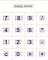image2-Basic Structure of calculator

according to this structure we can notice that the basic structure of calculator is build up as a table. the numbers and arithmetic exist in vertical and horizontal columns. as a example we can see 1,2,3 and — are into horizontal columns of a table. and 7,4,1 and 0 are into vertical columns of a table. so like that other numbers and things are include. so in the calculating process, we click two or more numbers and click arithmetic exist and then get the answer. in this case , in the html file format we can create this symbols as click buttons and they can be included in a table.

So firstly we add to html file format in to html. as we have discuss earlier how to add html form format , now i don’t talk about it. and then add attribute for form element “name” and calculator as a value.

`<form name=”calculator”>      </form>`

After that we need to add a table. we also know how to add table in html format.

`<table>   elements</table>`

after we insert the table we need to add columns and rows of table. in html format we know <tr> tag use to defined horizontal lines and <td> tags use to define vertical lines. so I don’t try to describe about table format in HTML. in this calculator format(image2) we can see the 1st row is use to display answer that we need to get by calculation. and then after 1st row, we can see there are 4 rows, they are buttons that we use to click. we need to add these buttons row by row to the table.

`<html>   <head>   <body>      <form name="calculator">         <table>            <tr>               <td colspan="4">                  <input type="text" name="display" id="display">               </td>            </tr>            <tr>               <td><input type="button" name="seven" value="7" onclick="calculator.display.value += '7'"></td>               <td><input type="button" name="eight" value="8" onclick="calculator.display.value += '8'"></td>               <td><input type="button" name="nine" value="9" onclick="calculator.display.value += '9'"></td>               <td><input type="button" class="operator" name="div" value="/" onclick="calculator.display.value += '/'"></td>            </tr>            <tr>               <td><input type="button" name="four" value="4" onclick="calculator.display.value += '4'"></td>               <td><input type="button" name="five" value="5" onclick="calculator.display.value += '5'"></td>               <td><input type="button" name="six" value="6" onclick="calculator.display.value += '6'"></td>               <td><input type="button" class="operator" name="times" value="x" onclick="calculator.display.value += '*'"></td>            </tr>            <tr>               <td><input type="button" name="one" value="1" onclick="calculator.display.value += '1'"></td>               <td><input type="button" name="two" value="2" onclick="calculator.display.value += '2'"></td>               <td><input type="button" name="three" value="3" onclick="calculator.display.value += '3'"></td>               <td><input type="button" class="operator" name="minus" value="-" onclick="calculator.display.value += '-'"></td>            </tr>            <tr>               <td><input type="button" id="clear" name="clear" value="c" onclick="calculator.display.value = ''"></td>               <td><input type="button" name="zero" value="0" onclick="calculator.display.value += '0'"></td>               <td><input type="button" name="doit" value="=" onclick="calculator.display.value = eval(calculator.display.value)"></td>               <td><input type="button" class="operator" name="plus" value="+" onclick="calculator.display.value += '+'"></td>            </tr>         </table>      </form>   </body>   </head></html>`

Here this is the code. now explain it. under the 1st <td> tag of the table , i use to display the values that we click in calculator buttons.

`<td colspan="4">                  <input type="text" name="display" id="display">               </td>`

and then other rows of table, i use to define buttons. in other words the numbers and symbols that we click in calculator.

`<td><input type="button" name="one" value="1" onclick="calculator.display.value += '1'"></td>`

in this code I use input type element, name attribute and value attribute, the value attribute is used to what would be display in the click the buttons. the next I use Onclick attribute. it’s defined what is run when a buttons click. in other words Onclick attribute tell the web browser about the what’s the value the button holds.

and also I use value+=, it use to add another value to the 1st value, in other words when we click number 2, and then click number 3 then they add i order to one by one, but without using +=, when we click number 2, and after click number 3, then number 2 is deleted.

we move to last row of the table, in the last row, related to ‘=’ sign i make some different. the in interpret value of input as JavaScript. when we click 5+2 as input, it evaluate as JavaScript and get the value.

that is the basic parts that I used. This is the my Basic calculator . and also we can add design it to by using CSS.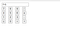This is the calculator that we build

So that’s the things that I have share with you. and I think you have got some knowledge about basic HTML form types and how to make a calculator. Thank you!

Have a nice day!!!

Undergraduate at University of Moratuwa

## More from sahan thathsara

Undergraduate at University of Moratuwa